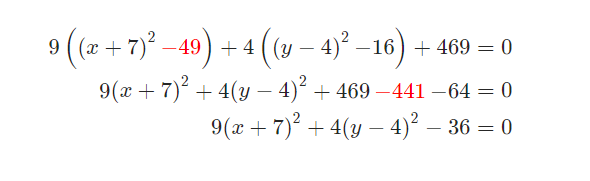### ¿Todavía tienes preguntas de matemáticas?

Pregunte a nuestros tutores expertos
Algebra
Pregunta

5. Complete the square on the $$x$$ and $$y$$ portions of the equation and write the equation into the standard form of the equation of the ellipse.

$9{x^2} + 126x + 4{y^2} - 32y + 469 = 0$

The process here will be very similar to the process we used in the previous section to write equations of parabolas in standard form. We’ll modify it slightly and also need to do an extra step or two but it is pretty similar to that process.

The first step is to make sure the coefficient of the $${x^2}$$ and $${y^2}$$ is a one. The $${x^2}$$ has a coefficient of 9 and the $${y^2}$$ has a coefficient of 4 and so we need to take care of each of those. What we will do is factor a 9 out of every term involving an $$x$$ and factor a 4 out of every term involving a $$y$$. Doing that gives,

$9\left( {{x^2} + 14x} \right) + 4\left( {{y^2} - 8y} \right) + 469 = 0$

Note that unlike the parabola work we don’t factor anything out of the constant term regardless of what we factor out of the $$x$$ and/or $$y$$ terms.

Show Step 2

Now let’s get started on completing the square. First, we need one-half the coefficient of the $$x$$ and $$y$$ term, square each and the add/subtract those numbers in the appropriate places as follows,

${\left( {\frac{{14}}{2}} \right)^2} = {\left( 7 \right)^2} = \space {\color{Red} 49 } \hspace{0.25in}{\left( {\frac{{ - 8}}{2}} \right)^2} = {\left( { - 4} \right)^2} = \space {\color{ProcessBlue} 16}$ $9\left( {{x^2} + 14x \space {\color{Red} + 49 - 49}} \right) + 4\left( {{y^2} - 8y \space {\color{ProcessBlue}+ 16 - 16} } \right) + 469 = 0$

Be careful you add/subtract these numbers and make sure you put them in the parenthesis!

Show Step 3

Next, we need to factor the $$x$$ and $$y$$ terms and add up all the constants.When adding the constants up, make sure to multiply the 9 through the $$x$$ terms and the 4 through the $$y$$ terms before adding the constants up.

Show Step 4

To finish things off we’ll first move the 36 to the other side of the equation.

$9\left( {{{\left( {x + 7} \right)}^2}} \right) + 4\left( {{{\left( {y - 4} \right)}^2}} \right) = 36$

To get this into standard form we need a one on the right side of the equation. To get this all we need to do is divide everything by 36 and we’ll do a little simplification work on the terms.

$\frac{{9\left( {{{\left( {x + 7} \right)}^2}} \right)}}{{36}} + \frac{{4\left( {{{\left( {y - 4} \right)}^2}} \right)}}{{36}} = 1\hspace{0.25in} \Rightarrow \hspace{0.25in}{{\frac{{{{\left( {x + 7} \right)}^2}}}{4} + \frac{{{{\left( {y - 4} \right)}^2}}}{9} = 1}}$

Solución
View full explanation on CameraMath App.